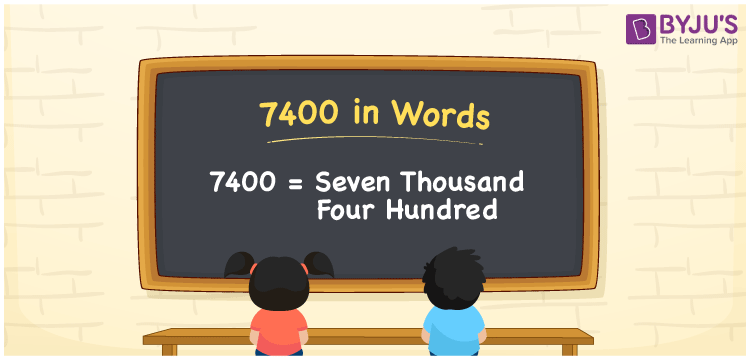# 7400 in words

7400 in words is written as Seven Thousand Four hundred. 7400 represents the count or value. The article on Counting Numbers can give you an idea about count or counting. The number 7400 is used in expressions that relate to money, distance, length and others. Let us consider an example for 7400. “The distance between India and Australia is around Seven Thousand Four Hundred kms.” Another example is, “Last night dinner at a restaurant cost us Seven Thousand Four Hundred Rupees”.

 7400 in words Seven Thousand Four Hundred Seven Thousand Four Hundred in Numbers 7400

## 7400 in English Words## How to Write 7400 in Words?

We can convert 7400 to words using a place value chart. The number 7400 has 4 digits, so let’s make a chart that shows the place value up to 4 digits.

 Thousands Hundreds Tens Ones 7 4 0 0

Thus, we can write the expanded form as:

7 × Thousand + 4 × Hundred + 0 × Ten + 0 × One

= 7 × 1000 + 4 × 100 + 0 × 10 + 0 × 1

= 7400

= Seven Thousand Four Hundred.

7400 is the natural number that is succeeded by 7399 and preceded by 7401.

7400 in words – Seven Thousand Four Hundred.

Is 7400 an odd number? – No.

Is 7400 an even number? – Yes.

Is 7400 a perfect square number? – No.

Is 7400 a perfect cube number? – No.

Is 7400 a prime number? – No.

Is 7400 a composite number? – Yes.

## Solved Example

1. Write the number 7400 in expanded form

Solution: 7 × 1000 + 4 × 100 + 0 × 10 + 0 × 1

We can write 7400 = 7000 + 400 + 0 + 0

= 7 × 1000 + 4 × 100 + 0 × 10 + 0 × 1

## Frequently Asked Questions on 7400 in words

Q1

### How to write 7400 in words?

7400 in words is written as Seven Thousand Four hundred.
Q2

### State True or False. 7400 is divisible by 2?

True. 7400 is divisible by 2.
Q3

### Is 7400 divisible by 10?

Yes. 7400 is divisible by 10.